Courses

# Power Electronics And Drives - MCQ Test 2

## 20 Questions MCQ Test Mock Test Series for Electrical Engineering (EE) GATE 2020 | Power Electronics And Drives - MCQ Test 2

Description
This mock test of Power Electronics And Drives - MCQ Test 2 for GATE helps you for every GATE entrance exam. This contains 20 Multiple Choice Questions for GATE Power Electronics And Drives - MCQ Test 2 (mcq) to study with solutions a complete question bank. The solved questions answers in this Power Electronics And Drives - MCQ Test 2 quiz give you a good mix of easy questions and tough questions. GATE students definitely take this Power Electronics And Drives - MCQ Test 2 exercise for a better result in the exam. You can find other Power Electronics And Drives - MCQ Test 2 extra questions, long questions & short questions for GATE on EduRev as well by searching above.
QUESTION: 1

### A 6-phase bridge-converter feeds a purely resistive load. The  delay angle α is measured from the point of naturalcommutation. The effective control of voltage can be obtained  when α lies in the range

Solution:

Refer 12-pulse bridge converter which
would behave as 6-phase bridge converter

QUESTION: 2

### A 3-phase cycloconverter is used to obtain  a variable frequency single-phase a.c. output. The single phase a.c. load  is 220 V, 60 A at a power factor of 0.6 lagging. The  rms value  of input voltage per phase required is

Solution: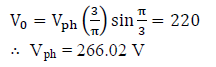QUESTION: 3

### In the below given circuit, the FD (Freewheeling diode) is forward biased at ωt =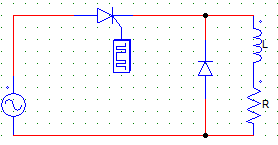Solution:

It is forward biased at π by the conducting SCR & the current starts to through the FD & Load.

QUESTION: 4

A d.c. source is switched in steps to synthesize the three-phase  output. The basic three-phase bridge inverter can be  controlled. The angle through which each switch conducts and  at any instant the number of switches conducting  simultaneously are, respectively

Solution:
QUESTION: 5

For a single-phase, full-bridge inverter supplying power to a  highly inductive load  as shown below, the correct sequence of operation of switches and diodes is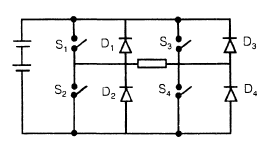Solution:
QUESTION: 6

single-phase, half-bridge inverter has input voltage of 48 V DC. Inverter is feeding a load of 2.4Ω. The r.m.s. output voltage at fundamental frequency is

Solution: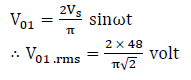QUESTION: 7

A boost-regulator has an input volage of 5V and the average utput voltage of 15V. The duty  cycle is

Solution:

Boost regulator is a step-up chopper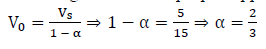QUESTION: 8

Which one of the following statements is The turn off times of converter grade SCRs are normally in  the range of

Solution:
QUESTION: 9

Which one of the following statements is

A Triac is a

Solution:
QUESTION: 10

Which one of the following is correct?

In a switched capacitor network for VAR compensation the SCRs are commutated by

Solution:
QUESTION: 11

Which one of the following shows current fold back  characteristics curve for a SCR controlled shunt regulated  power supply?

Solution:
QUESTION: 12

Which one of the following statements is correct? In a transistor, the reverse saturation current ICO

Solution:
QUESTION: 13

Which one of the following statements is correct. In a thyristor, the holding current IH  is

Solution:

IL = 2 to 3 times IH

QUESTION: 14

Which one of the following statements is correct ? For a SCR, dv/dt protection is achieved through the use of

Solution:
QUESTION: 15

In the buck-boost converter, what is the maximum value of the switch utilization - factor?

Solution:
QUESTION: 16

Which one of the following statements is correct?

In order to get best results per unit cost, the heat sinks on which the thyristors are mounted, are made of

Solution:
QUESTION: 17

Which one of the following is used as the main switching element in a switched mode power supply operating in 20 kHz to 100 kHz range?

Solution:

MOSFET has lowest switching losses and
operates at high frequencies (~KHz range).

QUESTION: 18

A converter which can operate both in 3-pulse and 6-pulse modes is a

Solution:

A 3-phase semiconverter has the unique
feature of working as a six-pulse converter
for α < 60° and as a three-pulse converter
for α ≥ 60°.

QUESTION: 19

A gate turn off (GTO) thyristor has capacity to

Solution:
QUESTION: 20

Match List-I (Limiting factor) with List-II (Safe Operating Area Portion) and select the correct answer using the codes given below the lists:

List-I

A. The peak voltage limit
B. Secondary breakdown limit
C. Power dissipation limit
D. Peak current limitList-II

1. PQ
2. QR
3. RS
4. ST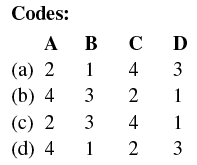Solution: